### 时间序列预测的顺序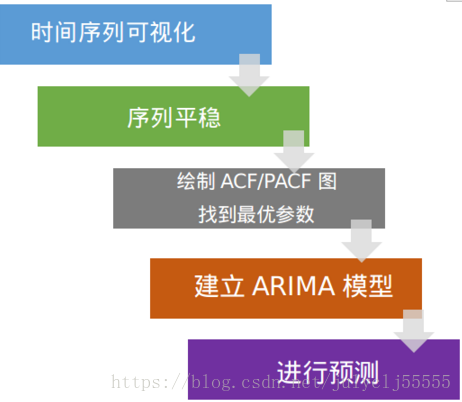### 1 其中常见的时间序列预测算法

#### 1.1 朴素预测法（一次指数平滑）

$X_{t+1} = X_i$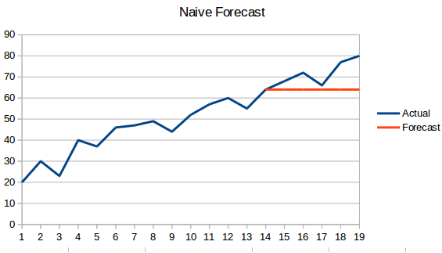#### 1.2 简单平均法

$X_{t+1} = \frac{1}{N} \sum^N_{i=1} X_i$

where:N表示所有的历史数据的总数.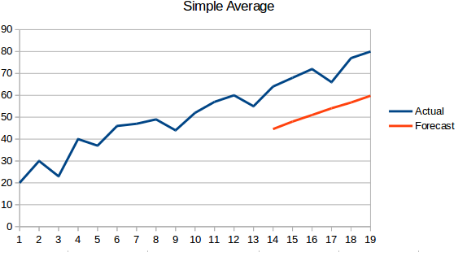#### 1.3 移动平均法

$X_{t+1} = \frac {1}{n} \sum^n_i X_i$

where:n表示前n个数据的数据总数#### 1.4 加权移动平均法

$X_{t+1} = \frac {1}{n} \sum^n_i X_i \cdot W_i$

where:W表示每个数据点的权重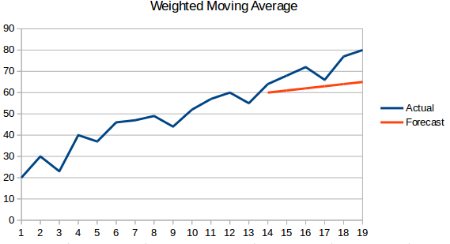### 1.5 简单指数平均法

$X_{t+1} = \frac {1}{n} \sum^n_i X_i \cdot W_i 且W_i>W_{i-1}$#### 1.6 霍尔特（Holt）线性趋势预测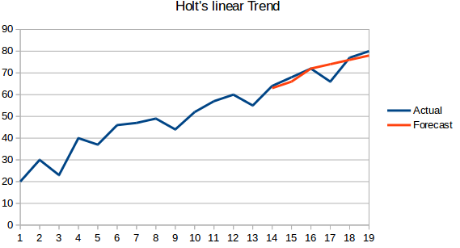#### 1.7 霍尔特-温特斯（Holt Winters）方法（三次指数平滑）#### 2.1 MSE-均方误差(mean square error)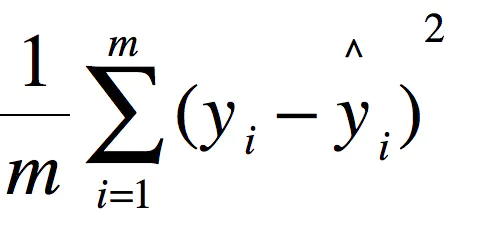#### 2.2 RMSE-均方根误差(root mean square error)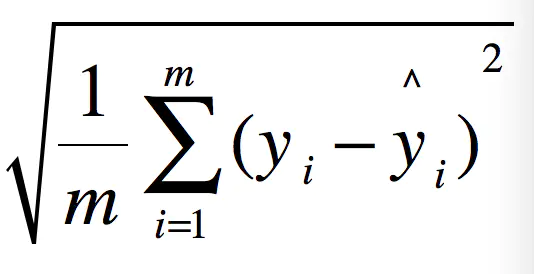#### 2.3 MAE-平均绝对误差(mean absolute error)#### 2.4 R Squared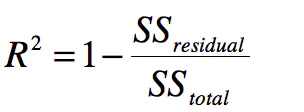• 上面分子就是我们训练出的模型预测的所有误差。
• 下面分母就是不管什么我们猜的结果就是y的平均数。（瞎猜的误差）

• 如果结果是0，就说明我们的模型跟瞎猜差不多。
• 如果结果是1。就说明我们模型无错误。
• 如果结果是0-1之间的数，就是我们模型的好坏程度。
• 如果结果是负数。说明我们的模型还不如瞎猜。（其实导致这种情况说明我们的数据其实没有啥线性关系）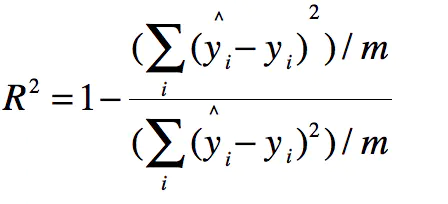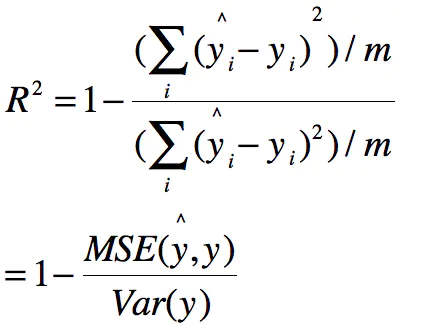### 3 循环神经网络RNN的结构如下：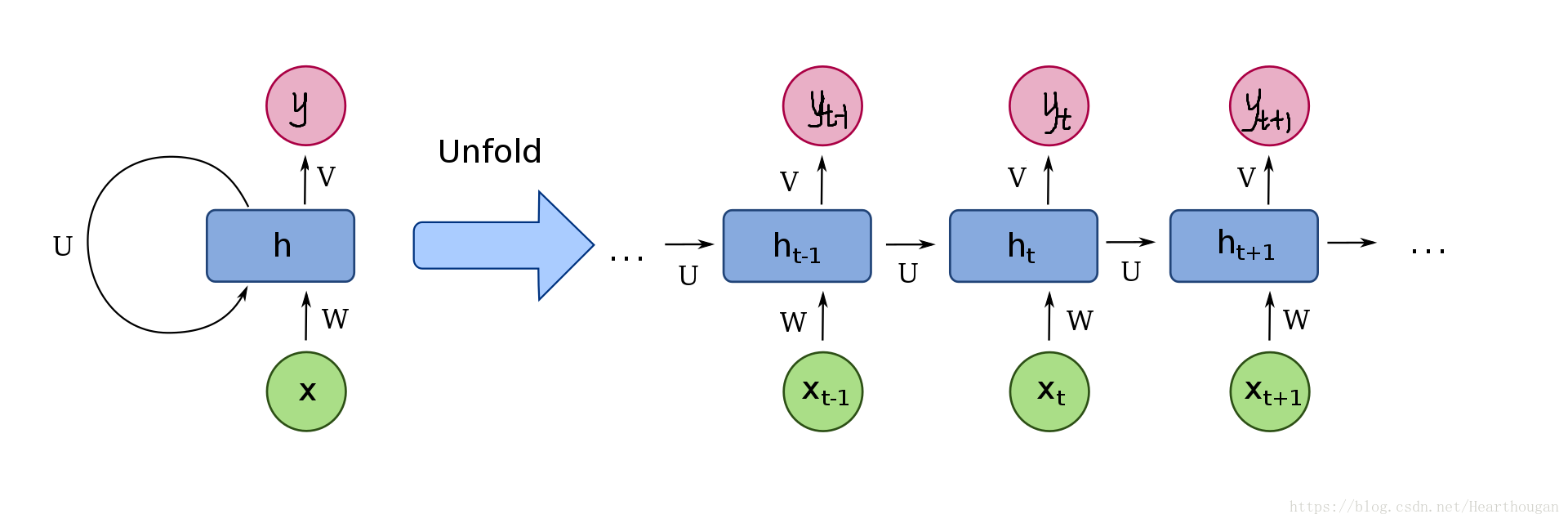#### （1） 梯度爆炸的解决方法

• 重新设计网络结构
• 使用激活函数（ReLU函数等）
• 使用权重正则化
• 使用梯度剪枝
• LSTM

#### （2） 梯度消失的解决办法

• GRU（Gate Recurrent Unit），门循环单元
• LSTM（Long Short Term Memory）,长短时记忆网络。

#### 3.1 长短时记忆网络

##### （1）LSTM介绍

• 一个LSTM Cell是由3个门限结构和1个状态向量传输线组成的，门限分别是遗忘门，传入门，输出门；
• 其中状态向量传输线负责长程记忆，因为它只做了一些简单的线性操作；3个门限负责短期记忆的选择，因为门限设置可以对输入向量做删除或者添加操作;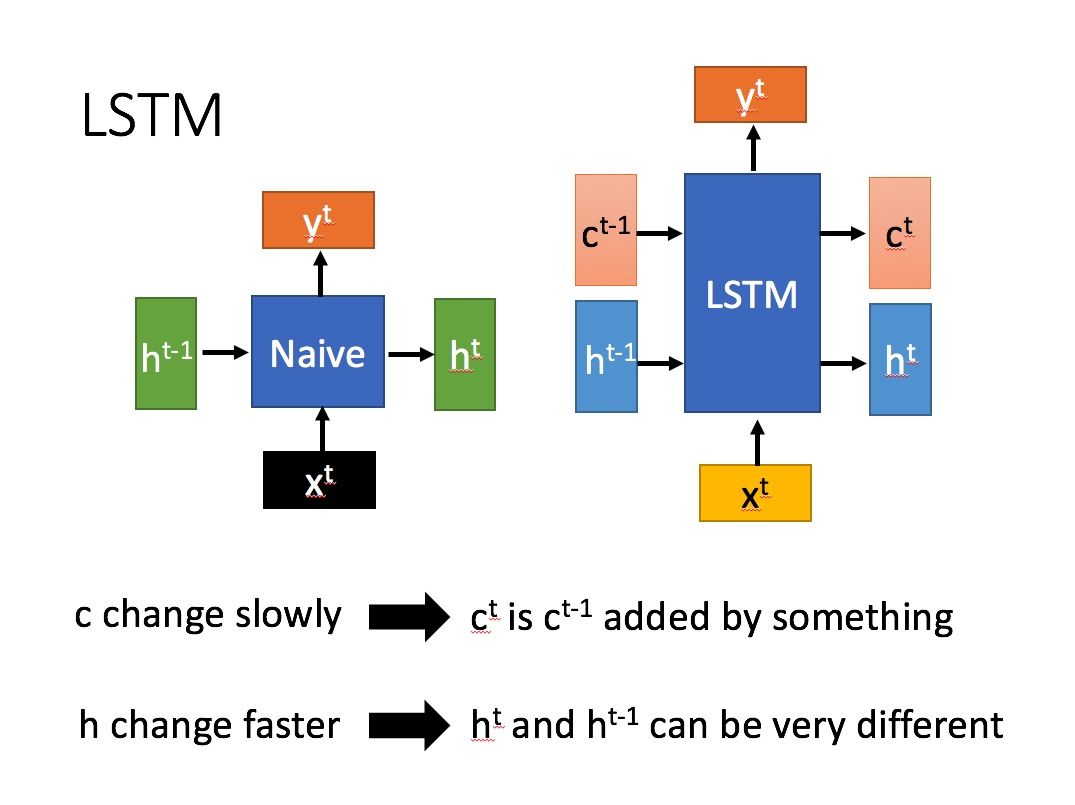LSTM中的参数介绍：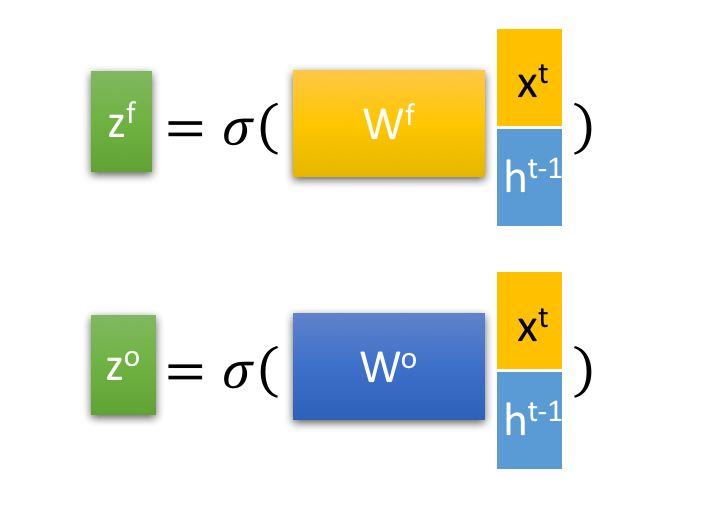LSTM的内部结构：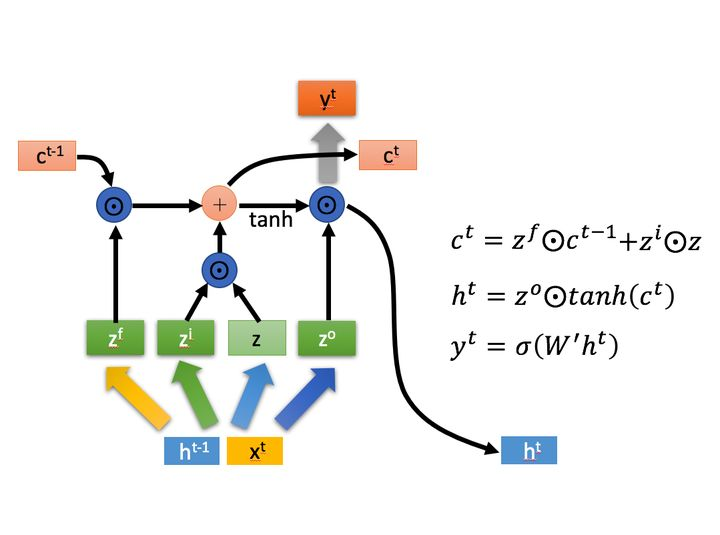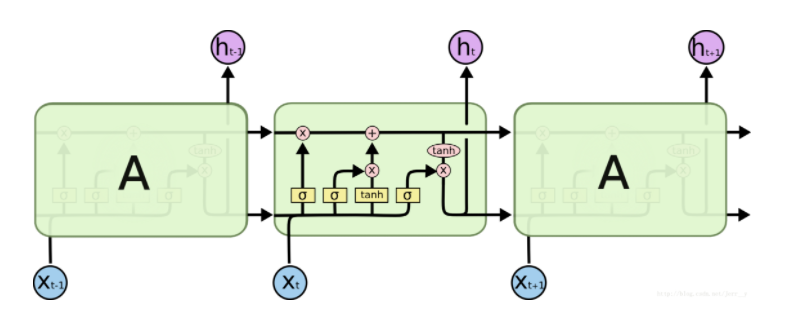1）遗忘门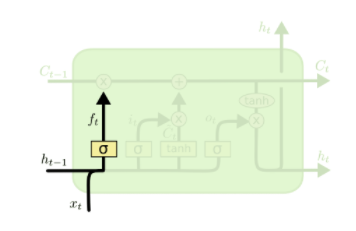2）输入门（选择记忆）3）输出门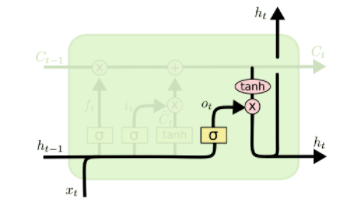4）状态更新

##### （2）LSTM的优缺点介绍
• 优点
• 解决了RNN中的梯度消失和梯度爆炸问题
• 缺点
• 计算速度较慢

#### 3.2 GRU

##### （1）GRU的结构介绍

GRU的内部结构图：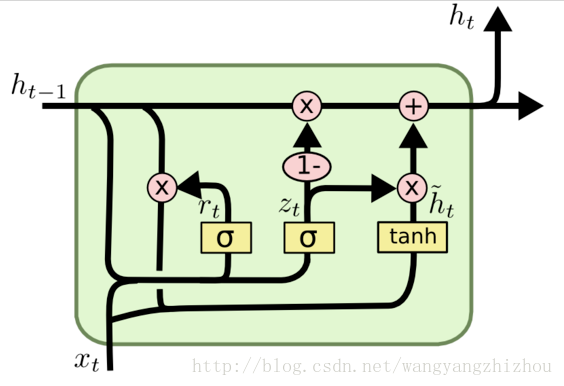GRU的状态图：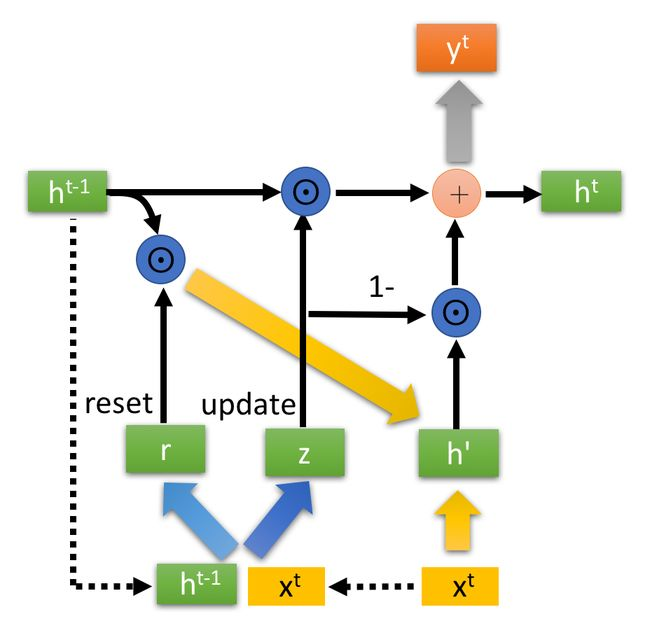GRU的两个门控：AR（p）,MA（q）

### 6 差分自回归移动平均（ARIMA）

AR是（Auto Regressive）自回归，p是自回归项；MA（Moving Average）是移动平均，q是移动平均项；d是时间序列成为平稳时所做的差分次数。
ARIMA模型的提出是为了解决ARMA模型不能预测非随机平稳过程的问题，ARIMA的思路是：现将给定的非平稳随机过程转换成平稳随机过程，然后再使用ARMA模型进行预测。

### 8 三次指数平滑

#### 8.1 为什么叫“指数平滑”？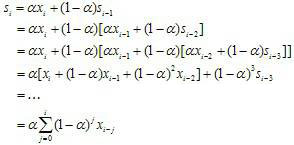#### 机器学习模型中的训练集、校验集、测试集

• 训练集：用于训练模型，找出最佳的w和b。
• 验证集：用以确定模型超参数，选出最优模型。
• 测试集：仅用于对训练好的最优函数进行性能评估。

### 写在最后